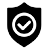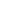#### WhatsApp Quick Chat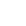#### Get Instant Call Back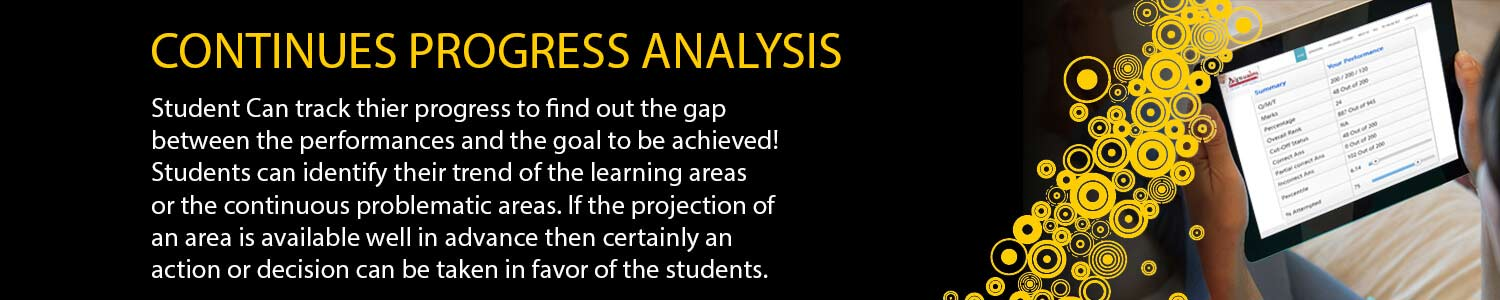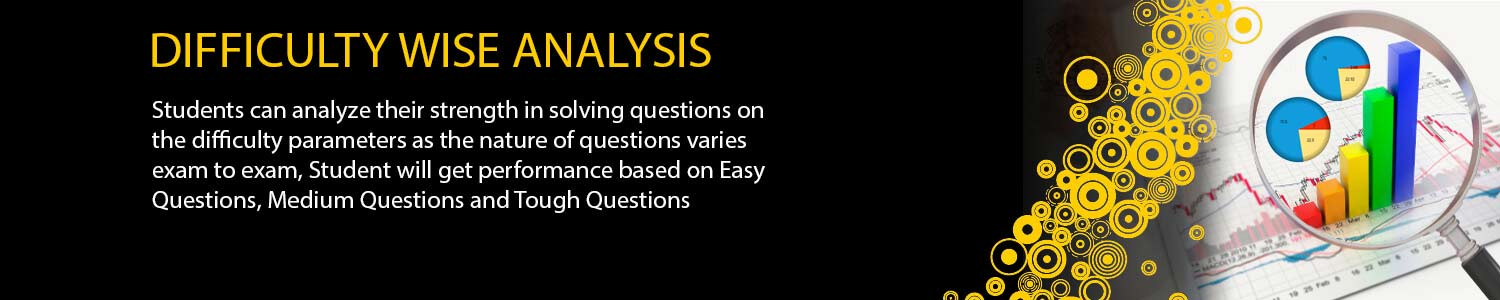## IIT JAM STAT

#### Offers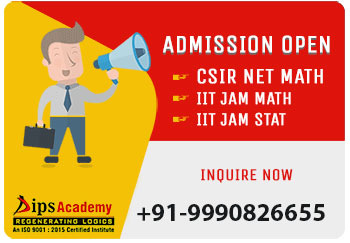##### News & Events# JAM Stat OTS Test Series Schedule

# DATE TEST TYPE MODULES IIT-JAM STATISTICSTEST SERIES 2021 05-Jan-21 MWT-01 Basic Probability and Random Variables Probability: Axiomatic definition of probability and properties, conditional probability, multiplication rule. Theorem of total probability. Bayes' theorem and independence of events.Random Variables: Probability mass function, probability density function and cumulative distribution functions, distribution of a function of a random variable. Mathematical expectation, moments and moment generating function. 08-Jan-21 MWT-02 Standard Distribution Standard Distributions: Binomial, negative binomial, geometric, Poisson, hypergeometric, uniform, exponential, gamma, beta and normal distributions. Poisson and normal approximations of a binomial distribution. Sampling distributions: Chi-square, t and F distributions, and their properties. 11-Jan-21 MWT-03 Joint Distributions Joint Distributions: Joint, marginal and conditional distributions. Distribution of functions of random variables. Joint moment generating function. Product moments, correlation, simple linear regression. Independence of random variables. 14-Jan-21 MWT-04 Estimation Estimation:Unbiasedness, consistency and efficiency of estimators, method of moments and method of maximum likelihood. Sufficiency, factorization theorem. Completeness, Rao-Blackwell and Lehmann-Scheffe theorems, uniformly minimum variance unbiased estimators. Rao-Cramer inequality. Confidence intervals for the parameters of univariate normal, two independent normal, and one parameter exponential distributions. 17-Jan-21 MWT-05 Testing of Hytothesis & Limit Theorem Limit Theorems: Weak law of large numbers. Central limit theorem (i.i.d.with finite variance case only).Chebyshev's inequality. Testing of Hytothesis: Basic concepts, applications of Neyman-Pearson Lemma for testing simple and composite hypotheses. Likelihood ratio tests for parameters of univariate normal distribution. Basic concepts, applications of Neyman-Pearson Lemma for testing simple and composite hypotheses. Likelihood ratio tests for parameters of univariate normal distribution. 20-Jan-21 MWT-06 Real Analysis Sequences and Series: Convergence of sequences of real numbers, Comparison, root and ratio tests for convergence of series of real numbers. Differential Calculus: Limits, continuity and differentiability of functions of one and two variables. Rolle's theorem, mean value theorems, Taylor's theorem, indeterminate forms, maxima and minima of functions of one and two variables. 23-Jan-21 MWT-07 Linear Algebra Matrices: Rank, inverse of a matrix. Systems of linear equations. Linear transformations, eigenvalues and eigenvectors. Cayley-Hamilton theorem, symmetric, skew-symmetric and orthogonal matrices. 26-Jan-21 MWT-08 Integral Calculus Integral Calculus: Fundamental theorems of integral calculus. Double and triple integrals, applications of definite integrals, arc lengths, areas and volumes. 29-Jan-21 FLT-01 Full Length Test As per Exam Pattern. 01-Feb-21 FLT-02 Full Length Test As per Exam Pattern. 04-Feb-21 FLT-03 Full Length Test As per Exam Pattern. 07-Feb-21 FLT-04 Full Length Test As per Exam Pattern.

## Offers## News & Events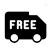##### Free Delivery